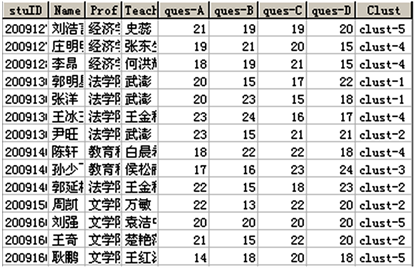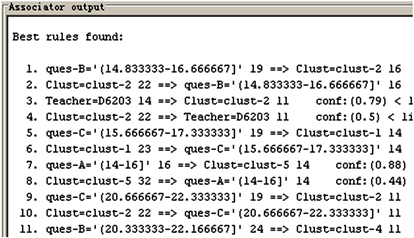# 聚类联合关联规则的数据挖掘技术The Combining Technology of Data Mining Based on Clustering and Association Rules

DOI: 10.12677/ORF.2017.74018, PDF, HTML, XML, 下载: 1,002  浏览: 2,418  科研立项经费支持

Abstract: Although clustering analysis and association rules as two main application methods can achieve data mining, but both two methods have three different. The data type of clustering operation is continuous and association rules are discrete. Clustering reflects the description function of the mining and association rules reflect prediction/validation function. The output form of clustering is clusters, and association rules then output the lines of rule. At the same time, both of them have some complementary to each other. So, this paper combined the both methods. The clustering analysis for the set of samples was first executed. This processing will make samples for their respective category entity information. Then, run association rules mining according to the samples what with classification properties. The method show the potential knowledge further including causes of the formation of clustering and the relationship between clusters. The experiment shows that the mining technology has better effect and great value of application.

1. 引言

2. 聚类联合关联规则的挖掘技术

2.1. 聚类分析

${S}_{t}=\underset{i=1}{\overset{{n}_{t}}{\sum }}{\left({x}_{it}-{\stackrel{¯}{x}}_{t}\right)}^{\prime }\left({x}_{it}-{\stackrel{¯}{x}}_{t}\right)$

$S=\underset{t=1}{\overset{k}{\sum }}{S}_{t}=\underset{t=1}{\overset{k}{\sum }}\underset{i=1}{\overset{n}{\sum }}{\left({x}_{it}-{\stackrel{¯}{x}}_{t}\right)}^{\prime }\left({x}_{it}-{\stackrel{¯}{x}}_{t}\right)$

2.2. 关联规则

“可能性比较高”的界定方法，则采用支持度和置信度来表述：

L={large 1-itemsets};

for (k=2; L[k-1]≠Φ; k=k+1) do

C[k]=apriori_gen(L[k-1]); //构造候选项集

for all transactions t∈D do

C[t]=subset(C[k], t);

//搜索事务t中包含的候选项集

for all C∈C[t] do C.sup=C.sup+1; end for

//计算支持数

end for

L[k]={ C∈C[k] | C.sup>=minsup};

//得到K阶大项集

end for

L=U[k] L[k];

insert into C[k]

select P, P, ∙∙∙, P[k − 1], Q[k − 1]

from L[k − 1] P, L[k − 1] Q

where P= Q, ∙∙∙, P[k − 2] = Q[k − 2], P[k − 1] < Q[k − 1]

for all itemsets C Î C[k] do

for all (k − 1) itemsets S of C do

if (SÏL[k − 1]) then delete C from C[k]

2.3. 联合运用

3. 实验数据与方法

3.1. 样本数据Table 1. Function contrast of clustering and association rule

3.2. 数据变换

3.3. 聚类分析

3.4. 关联规则挖掘

4. 结果与讨论Figure 1. Cluster analysisFigure 2. Data mining results of association rules after clusteringTable 3. Clustering results analysis of ample data

ques-B = 14.8-16. 7 ==> Clust = clust-2

Clust = clust-2 ==> Teacher = D6203

5. 结语

NOTES



*通讯作者。

  陈安, 陈宁, 周龙骧. 数据挖掘技术及应用[M]. 北京: 科学出版社, 2006.  Agrawal, R., Imielinski, T. and Swami, A. (1993) Database Mining: A Performance Perspective. IEEE Transactions on Knowledge and Data Engineering, 5, 914-925. https://doi.org/10.1109/69.250074  夏姜虹. 数据挖掘技术的常用方法分析[J]. 云南大学学报(自然科学版), 2011, 33(S2): 173-175.  张连育, 吕立. 基于策略模式的中医数据挖掘平台的设计与研究[J]. 小型微型计算机系统, 2011, 32(7): 1406- 1411.  孙中祥, 彭湘君, 杨玉平, 贺一. 数据挖掘在教育教学中的应用综述[J]. 2012, 2(1): 78-80.  戴汝为. 社会智能科学[M]. 上海: 上海交通大学出版社, 2007.  张东生, 王永强, 苏靖, 等. 模糊聚类与数据挖掘在数据分析中的应用[J]. 运筹与模糊学, 2016, 6(4): 7  Agrawal, R. and Srikant, R. (1995) Mining Sequential Patterns. 1995 Proceedings of the Eleventh International Conference on Data Engineering, Taipei, 6-10 March 1995, 3-14. https://doi.org/10.1109/ICDE.1995.380415  张东生. 基于模糊聚类的考试分析方法[J]. 电脑知识与技术, 2009, 5(33): 9579-9580.  李雪梅, 张素琴. 数据挖掘中聚类分析技术的应用[J]. 武汉大学学报(工学版), 2009, 42(3): 396-399.  徐辉增. 关联规则数据挖掘方法的研究[J]. 科学技术与工程, 2012, 12(1): 60-63.  王爱平, 王占凤, 陶嗣干, 等. 数据挖掘中常用关联规则挖掘算法[J]. 计算机技术与发展, 2010, 20(4): 105-108.  张东生, 季超. 动态模糊聚类及最佳聚类效果研究[C]. Proceedings of Chinese Conference on Pattern Recognition (CCPR), Beijing, 4-6 November 2009.  Zhang, D.S., Li, S.Z. and Wei, W. (2010) Visual Clustering Methods with Feature Displayed Function for Self-Organizing. Industrial Mechatronics and Automation. https://doi.org/10.1109/ICINDMA.2010.5538274  郭涛, 张代远. 基于关联规则数据挖掘Apriori算法的研究与应用[J]. 计算机技术与发展, 2011, 21(6): 101-103.  武森, 俞晓莉, 倪宇, 王瑞峰. 数据挖掘中的聚类技术在学生成绩分析中的应用[J]. 中国管理信息化, 2009, 12(15): 45-47.